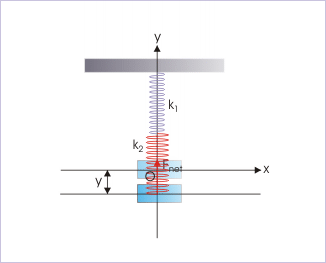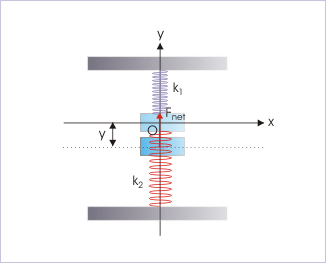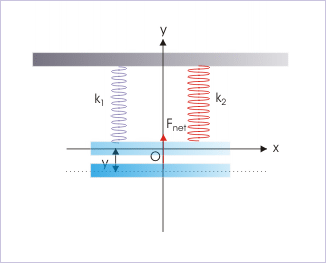# 1.7 Block – spring system in shm  (Page 2/2)

 Page 2 / 2

$⇒{F}_{\text{net}}=ma=-ky$

$⇒a=-\frac{k}{my}$

This relation on comparison with SHM equation “ $a=-{\omega }^{2}x$ ” yields same set of periodic expressions as in the case of horizontal block-spring arrangement :

$⇒\omega =\sqrt{\left(\frac{k}{m}\right)}\phantom{\rule{1em}{0ex}}⇒T=2\pi \sqrt{\left(\frac{m}{k}\right)}\phantom{\rule{1em}{0ex}}⇒\nu =\frac{1}{2\pi }\sqrt{\left(\frac{k}{m}\right)}$

In this case, however, we can obtain alternative expressions as well for the periodic attributes as spring force at equilibrium position is equal to the weight of the block,

$mg=-k{y}_{0}$

Dropping negative sign and rearranging,

$⇒\frac{m}{k}=\frac{{y}_{0}}{g}$

Hence, the alternative expressions of periodic attributes are :

$⇒\omega =\sqrt{\left(\frac{g}{{y}_{0}}\right)}\phantom{\rule{1em}{0ex}}⇒T=2\pi \sqrt{\left(\frac{{y}_{0}}{g}\right)}\phantom{\rule{1em}{0ex}}⇒\nu =\frac{1}{2\pi }\sqrt{\left(\frac{g}{{y}_{0}}\right)}$

Clearly, the extension of the spring owing to the weight of the block in vertical orientation has no impact on the periodic attributes of the SHM. One important difference, however, is that the center of oscillation does not correspond to the position of neutral spring configuration; rather it is shifted down by a vertical length given by :

$⇒{y}_{0}=\frac{mg}{k}$

## Block connected to springs in series

We consider two springs of different spring constants. An external force like gravity produces elongation in both springs simultaneously. Since spring is mass-less, spring force is same everywhere in two springs. This force, however, produces different elongations in two springs as stiffness of springs are different. Let “ ${y}_{1}$ ” and “ ${y}_{2}$ ” be the elongations in two springs. As discussed for the single spring, the net restoring force for each of the springs is given as :

${F}_{\text{net}}=-{k}_{1}{y}_{1}=-{k}_{2}{y}_{2}$The spring is stretched a bit from the equilibrium position and then let go to oscillate.

The total displacement of the block from equilibrium position is :

$⇒y={y}_{1}+{y}_{2}=-\frac{{F}_{\text{net}}}{{k}_{1}}-\frac{{F}_{\text{net}}}{{k}_{2}}$

$⇒{F}_{\text{net}}=\frac{{k}_{1}{k}_{2}y}{{k}_{1}+{k}_{2}}$

A comparison with the expression of extension of the single spring at equilibrium position reveals that spring constant of the arrangement of two springs is equivalent to a single spring whose spring constant is given by :

$⇒k=\frac{{k}_{1}{k}_{2}}{{k}_{1}+{k}_{2}}$

This relationship can also be expressed as :

$⇒\frac{1}{k}=\frac{1}{{k}_{1}}+\frac{1}{{k}_{2}}$

In the nutshell, we can consider the arrangement of two springs in series as a single spring of spring constant “k”, which is related to individual spring constants by above relation. Further, we can extend this concept to a number of springs by simply extending the relation as :

$⇒\frac{1}{k}=\frac{1}{{k}_{1}}+\frac{1}{{k}_{2}}+\frac{1}{{k}_{3}}+\dots$

The periodic attributes are given by the same expressions, which are valid for oscillation of single spring. We only need to use equivalent spring constant in the expression.

## Block in between two springs

In this arrangement, block is tied in between two springs as shown in the figure. In order to analyze oscillation, we consider oscillation from the reference position of equilibrium. Let the block is displaced slightly in downward direction (reasoning is similar if block is displaced upward). The upper spring is stretched, whereas the lower spring is compressed. The spring forces due to either of the springs act in the upward direction. The net downward displacement is related to net restoring force as :

${F}_{\text{net}}=-{k}_{1}{y}_{1}-{k}_{2}{y}_{2}=-\left({k}_{1}+{k}_{2}\right)y$The spring is stretched a bit from the equilibrium position and then let go to oscillate.

A comparison with the expression of extension of the single spring reveals that spring constant of the arrangement of two springs is equivalent to a single spring whose spring constant is given by :

$⇒k={k}_{1}+{k}_{2}$

Clearly, the periodic attributes are given by the same expressions, which are valid for oscillation of single spring. We only need to use equivalent spring constant in the expression.

## Block connected to springs in parallel

Here, we consider a block is suspended horizontally with the help of two parallel springs of different spring constants as shown in the figure. When the block is pulled slightly, it oscillates about the equilibrium position. The net restoring force on the block is :

${F}_{\text{net}}=-{k}_{1}{y}_{1}-{k}_{2}{y}_{2}=-\left({k}_{1}+{k}_{2}\right)y$The spring is stretched a bit from the equilibrium position and then let go to oscillate.

A comparison with the expression of extension of the single spring reveals that spring constant of the arrangement of two springs is equivalent to a single spring whose spring constant is given by :

$⇒k={k}_{1}+{k}_{2}$

Again, the periodic attributes are given by the same expressions, which are valid for oscillation of single spring. We only need to use equivalent spring constant in the expression.

what are the products of Nano chemistry?
There are lots of products of nano chemistry... Like nano coatings.....carbon fiber.. And lots of others..
learn
Even nanotechnology is pretty much all about chemistry... Its the chemistry on quantum or atomic level
learn
Preparation and Applications of Nanomaterial for Drug Delivery
Application of nanotechnology in medicine
what is variations in raman spectra for nanomaterials
I only see partial conversation and what's the question here!
what about nanotechnology for water purification
please someone correct me if I'm wrong but I think one can use nanoparticles, specially silver nanoparticles for water treatment.
Damian
yes that's correct
Professor
I think
Professor
what is the stm
is there industrial application of fullrenes. What is the method to prepare fullrene on large scale.?
Rafiq
industrial application...? mmm I think on the medical side as drug carrier, but you should go deeper on your research, I may be wrong
Damian
How we are making nano material?
what is a peer
What is meant by 'nano scale'?
What is STMs full form?
LITNING
scanning tunneling microscope
Sahil
how nano science is used for hydrophobicity
Santosh
Do u think that Graphene and Fullrene fiber can be used to make Air Plane body structure the lightest and strongest. Rafiq
Rafiq
what is differents between GO and RGO?
Mahi
what is simplest way to understand the applications of nano robots used to detect the cancer affected cell of human body.? How this robot is carried to required site of body cell.? what will be the carrier material and how can be detected that correct delivery of drug is done Rafiq
Rafiq
if virus is killing to make ARTIFICIAL DNA OF GRAPHENE FOR KILLED THE VIRUS .THIS IS OUR ASSUMPTION
Anam
analytical skills graphene is prepared to kill any type viruses .
Anam
Any one who tell me about Preparation and application of Nanomaterial for drug Delivery
Hafiz
what is Nano technology ?
write examples of Nano molecule?
Bob
The nanotechnology is as new science, to scale nanometric
brayan
nanotechnology is the study, desing, synthesis, manipulation and application of materials and functional systems through control of matter at nanoscale
Damian
Is there any normative that regulates the use of silver nanoparticles?
what king of growth are you checking .?
Renato
What fields keep nano created devices from performing or assimulating ? Magnetic fields ? Are do they assimilate ?
why we need to study biomolecules, molecular biology in nanotechnology?
?
Kyle
yes I'm doing my masters in nanotechnology, we are being studying all these domains as well..
why?
what school?
Kyle
biomolecules are e building blocks of every organics and inorganic materials.
Joe
anyone know any internet site where one can find nanotechnology papers?
research.net
kanaga
sciencedirect big data base
Ernesto
Got questions? Join the online conversation and get instant answers!By Cath YuBy Steve GibbsBy Katy PrattBy Mahee BooBy OpenStaxBy Madison ChristianBy Jonathan LongBy Carly AllenBy OpenStaxBy OpenStax